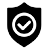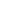#### WhatsApp Quick Chat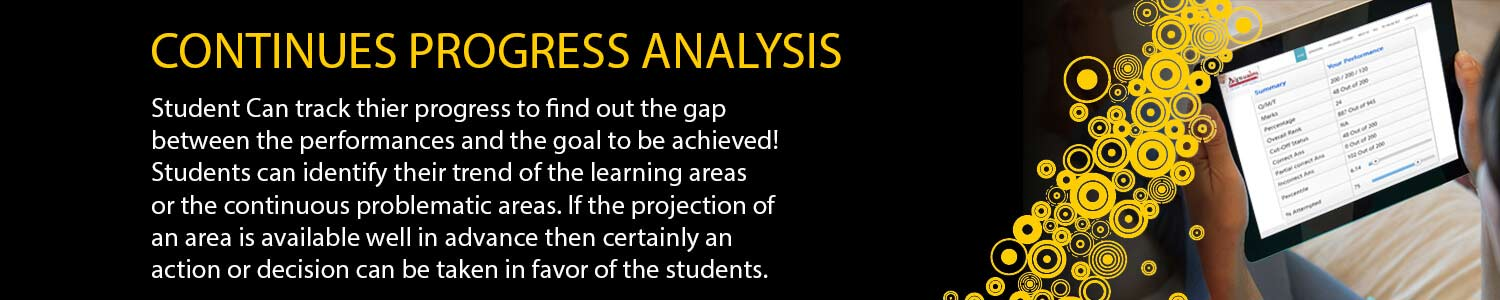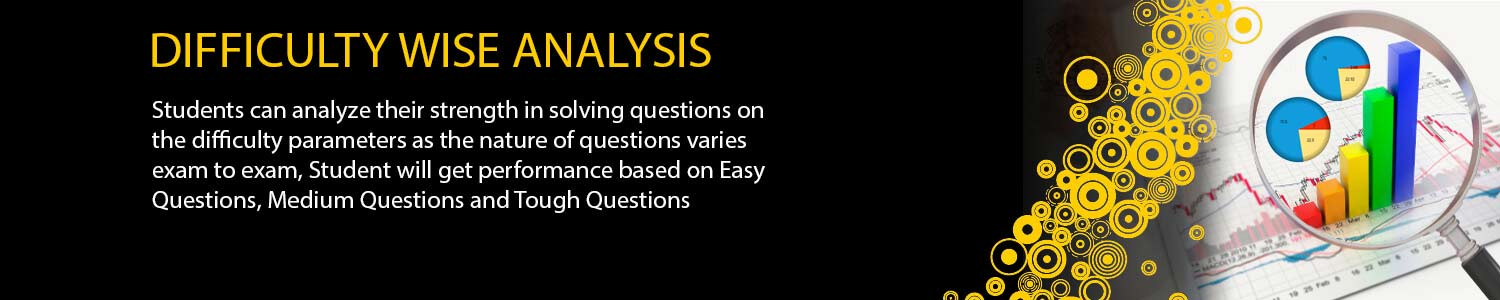## GATE STAT

#### Offers##### News & Events##### OTS (ONLINE TEST SERIES)
# DATE TEST TYPE MODULES Test Series ScheduleGate Statistics Test Series 2019 12 Jan 2019 MWT-01 Basic Probability Probability: Classical, relative frequency and axiomatic definitions of probability, conditional probability, Bayes’ theorem, independent events; Random variables and probability distributions, moments and moment generating functions, quantiles; Standard discrete and continuous univariate distributions; Probability inequalities (Chebyshev, Markov, Jensen); Function of a random variable. 14 Jan 2019 MWT-02 Joint Distribution Jointly distributed random variables, marginal and conditional distributions, product moments, joint moment generating functions, independence of random variables; Transformations of random variables, sampling distributions, distribution of order statistics and range; Characteristic functions; Modes of convergence; Weak and strong laws of large numbers; Central limit theorem for i.i.d. random variables with existence of higher order moments. 15 Jan 2019 MWT-03 Stochastic Process Stochastic Processes: Markov chains with finite and countable state space, classification of states, limiting behaviour of n-step transition probabilities, stationary distribution, Poisson and birth-and-death processes. 17 Jan 2019 MWT-04 Inference Confidence intervals; Tests of hypotheses, most powerful and uniformly most powerful tests, likelihood ratio tests, large sample test, Sign test, Wilcoxon signed rank test, MannWhitney U test, test for independence and Chi-square test for goodness of fit. 19 Jan 2019 MWT-05 Inference Vector spaces, subspaces, linear dependence, basis, dimension, algebra of linear transformations, matrix representation of linear transformation, Algebra of matrices, rank and determinant of matrices, system of linear equations. 20 Jan 2019 MWT-06 Regeression Analysis Simple and multiple linear regression, polynomial regression, estimation, confidence intervals and testing for regression coefficients; Partial and multiple correlation coefficients. 21 Jan 2019 MWT-07 Mutivariate Analysis Multivariate Analysis: Basic properties of multivariate normal distribution; Multinomial distribution; Wishart distribution; Hotellings T2 and related tests; Principal component analysis; Discriminant analysis; Clustering. 23 Jan 2019 MWT-08 Design of Experiment Design of Experiments: One and two-way ANOVA, CRD, RBD, LSD, 22 and 23 Factorial experiments. 25 Jan 2019 MWT-09 Mathematices Linear Algebra: Finite dimensional vector spaces over real or complex fields; Linear transformations and their matrix representations, rank; systems of linear equations, eigenvalues and eigenvectors, minimal polynomial, Cayley-Hamilton Theorem, diagonalization, Jordan canonical form, symmetric, skew-symmetric, Hermitian, skewHermitian, orthogonal and unitary matrices; Finite dimensional inner product spaces, Gram-Schmidt orthonormalization process, definite forms. Calculus: Finite, countable and uncountable sets, Real number system as a complete ordered field, Archimedean property; Sequences and series, convergence; Limits, continuity, uniform continuity, differentiability, mean value theorems; Riemann integration, Improper integrals; Functions of two or three variables, continuity, directional derivatives, partial derivatives, total derivative, maxima and minima, saddle point, method of Lagrange’s multipliers; Double and Triple integrals and their applications; Line integrals and Surface integrals, Green’s theorem, Stokes’ theorem, and Gauss divergence theorem. 27 Jan 2019 FLT-01 Full Length Test As per Exam Pattern. 29 Jan 2019 FLT-02 Full Length Test As per Exam Pattern. 31 Jan 2019 FLT-03 Full Length Test As per Exam Pattern.

## Offers## News & Events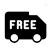##### Free Delivery